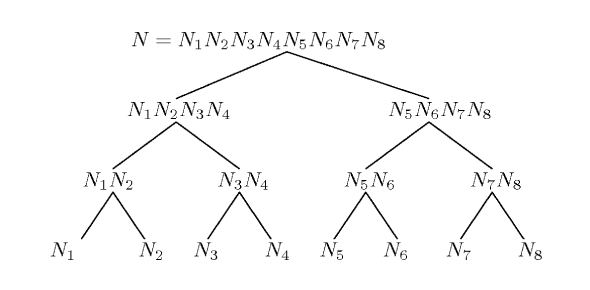# Computational Number Quiz! Test

Approved & Edited by ProProfs Editorial Team
At ProProfs Quizzes, our dedicated in-house team of experts takes pride in their work. With a sharp eye for detail, they meticulously review each quiz. This ensures that every quiz, taken by over 100 million users, meets our standards of accuracy, clarity, and engagement.
| Written by Livyn
L
Livyn
Community Contributor
Quizzes Created: 348 | Total Attempts: 140,973
Questions: 10 | Attempts: 37Settings.

• 1.

### Computational number theory is studied in .....

• A.

Mathematics

• B.

History

• C.

Language

• D.

Philosophy

A. Mathematics
Explanation
Computational number theory is a branch of mathematics that focuses on the study of algorithms and computational methods for solving problems in number theory. It involves using computers and mathematical software to analyze and solve complex number theory problems. This field combines the theoretical aspects of number theory with practical applications in computer science. Therefore, it is clear that computational number theory is studied in the field of mathematics.

Rate this question:

• 2.

### It is a subject also studied in .....

• A.

Languages

• B.

Computer science

• C.

History

• D.

Philosophy

B. Computer science
Explanation
Computer science is the subject that is also studied in languages, history, and philosophy. This means that computer science is a multidisciplinary field that intersects with various other disciplines. It involves the study of algorithms, programming languages, data structures, and the theory behind computing. It also explores the historical and philosophical aspects related to the development and impact of computers. Therefore, computer science is not limited to just one field but has connections and applications in different areas of study.

Rate this question:

• 3.

### Computational number theory is also known as ........

• A.

Algorithmic number theory

• B.

Transcendental number theory

• C.

Combinatorial number theory

• D.

Analytic number theory

A. Algorithmic number theory
Explanation
Computational number theory is a branch of mathematics that focuses on the development and implementation of algorithms to solve problems related to number theory. It involves the use of computer algorithms to study and analyze various number theoretic problems, such as prime factorization, modular arithmetic, and integer factorization. Therefore, the term "algorithmic number theory" accurately describes this field of study.

Rate this question:

• 4.

### On ....... Agrawal, Saxena, and Kayal found a polynomial time algorithm for testing and proving the primality of general numbers.

• A.

August 6, 2002

• B.

June 13, 1997

• C.

March 24, 2003

• D.

March 12, 2009

A. August 6, 2002
• 5.

### August 6, 2002, who found a polynomial time algorithm for testing and proving the primality of general numbers?

• A.

Agrawal, Saxena and Kayal

• B.

Isaac, John and James

• C.

Leibniz, Euler and Knuth

• D.

Kate, John and Isaac

A. Agrawal, Saxena and Kayal
Explanation
Agrawal, Saxena, and Kayal found a polynomial time algorithm for testing and proving the primality of general numbers on August 6, 2002.

Rate this question:

• 6.

### It is a course studied in.......

• A.

Mathematics

• B.

Sociology

• C.

Music

• D.

History

A. Mathematics
Explanation
The given options suggest different subjects in which the course could be studied. The correct answer is Mathematics, as it is a subject that commonly includes various courses and topics related to numbers, calculations, equations, and problem-solving.

Rate this question:

• 7.

### It is also a course studied in.....

• A.

Philosophy

• B.

Music

• C.

Computer Science

• D.

History

C. Computer Science
Explanation
Computer Science is the correct answer because it is a course that is studied in various academic disciplines such as Philosophy, Music, and History. However, Computer Science is specifically known for its focus on the study of computers, algorithms, programming languages, and the theory behind computational systems. It involves the exploration of various computer-related topics, including software development, data structures, artificial intelligence, and computer networking. Therefore, Computer Science is the most appropriate option among the given choices.

Rate this question:

• 8.

### Algorithmic number theory is the same thing as.....

• A.

Combinatorial number theory

• B.

Analytic number theory

• C.

Computational number theory

• D.

Transcendental number theory

C. Computational number theory
Explanation
Computational number theory refers to the study of algorithms and computational methods in number theory. It involves the use of computers and computational techniques to solve problems and explore properties of numbers. This is in contrast to other branches of number theory such as combinatorial number theory, which focuses on combinatorial methods and techniques, and analytic number theory, which deals with the use of complex analysis and analytic techniques in number theory. Transcendental number theory, on the other hand, specifically focuses on the properties and study of transcendental numbers. Therefore, computational number theory is the most appropriate option that aligns with algorithmic number theory.

Rate this question:

• 9.

### ...... the study of the algorithm for performing number-theoretic computations.

• A.

Computational number theory

• B.

Analytic number theory

• C.

Combinatorial number theory

• D.

Transcendental number theory

A. Computational number theory
Explanation
Computational number theory is the study of algorithms used to perform number-theoretic computations. This field focuses on developing efficient methods for solving problems related to prime numbers, factorization, modular arithmetic, and other number-theoretic concepts. It involves the use of computer algorithms and programming to analyze and solve mathematical problems in number theory. Analytic number theory, on the other hand, deals with the properties of numbers using methods from analysis, while combinatorial number theory focuses on the combinatorial properties of integers. Transcendental number theory studies the properties of transcendental numbers. Therefore, computational number theory is the most appropriate choice for the given definition.

Rate this question:

• 10.

### Computational number theory can be taught in how many courses?

• A.

1

• B.

2

• C.

3

• D.

4Back to top